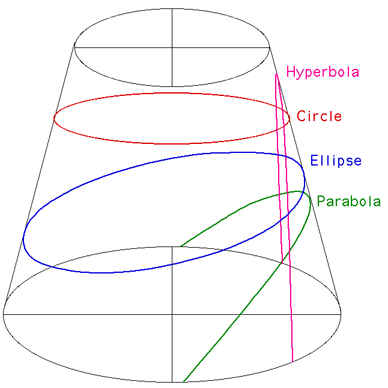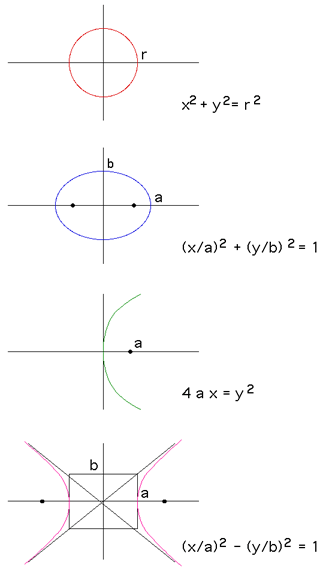# Conic Sections

Written by Paul Bourke
June 2002

If a cylinder is sliced by a plane a number of curves arise depending on the angle of the plane with respect to the cylinder axis, these are called conic sections. Conic sections were studied extensively by the Greeks as early as 350 BC in an attempt to solve the great geometric problems of the day, namely, squaring the circle, duplicating the cube, and trisecting an angle. They were also studied extensively in relation to Keplers laws of planetary motion by Descartes and Fermat. There are four different classes of curve (circle, ellipse, parabola, and hyperbola) and they are illustrated below.The equations for each of the curves types (centered at the origin) are given on the right. Note that the equations for the circle and ellipse are not dependent on the axis, the parabola and hyperbola come in two variants depending on whether they are aligned vertically or horizontally. Nappes If the cone is imagined to extend until it goes to a point and then enlarges again then the two cones are known as "nappes". A plane that intersects just one nappe is either an ellipse or parabola, if it intersects both nappes then it is a hyperbola. Focus The solid dots on the right indicate the focus of each curve. For the ellipse the focus distance is a2 - b2 and for the hyperbola it is a2 + b2. General equation The general equation of a conic section is given below. A x2 + B x y + C y2 + D x + E y + F = 0 The type of curve is dictated by the quantity B2 - 4 A C. If it is less than 0 the curve is an ellipse or circle. If equal to 0 then a parabola, if greater than 0 then a hyperbola.